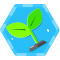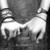# 一、this

`this` 是什么？其实它本身就是一种指向。`this` 指向可以分为以下几种情况

• 普通调用，`this` 指向为调用者
• `call/apply` 调用，`this` 指向为当前 `thisArg` 参数
• 箭头函数，`this` 指向为当前函数的 `this` 指向

## 1、普通调用

### 1.1、对象方法的调用

``````var obj = {
a: 'this is obj',
test: function () {
console.log(this.a);
}
}
obj.test();
// this is obj
``````

### 1.2、“单纯”函数调用

``````var a = 'this is window'
function test () {
console.log(this.a);
}
test();
// this is window
``````

``````window.a = 'this is window'
window.test = function test () {
console.log(this.a);
// 此时是window为调用者，即this会指向window
}
window.test();
``````

### 1.3、构造函数调用

``````function test () {
this.a = 'this is test';
console.log(this.a);
console.log(this);
}
test();
// this is test
// Window {}
``````

``````function Test () {
this.a = 'this is test';
console.log(this.a);
console.log(this);
}
var test = new Test();
// this is test
// Test {a: 'this is test'}
``````

OK，好像的确没有问题了，此时的 `this` 的确指向了该构造函数的实例对象。具体这里的一些解释后面我会在原型链继承里面详细讲解。

## 2、call/apply 调用

### 2.1、call 调用

`call` 方法形式，`fun.call(thisArg\[, arg1\[, arg2\[, ...\]\]\])`

• `thisArg`，当前 `this` 指向
• `arg1\[, arg2\[, ...\]\]`，指定的参数列表

``````function Test () {
this.a = 'this is test';
console.log(this.a);
console.log(this);
}
function Test2 () {
Test.call(this);
}
var test = new Test2();
// this is test
// Test2 {a: 'this is test'}
``````

### 2.2、apply 调用

`call` 类似，唯一的一个明显区别就是 `call` 参数为多个，`apply` 参数则为两个，第二个参数为数组或类数组形式，`fun.apply(thisArg, \[argsArray\])`

• `thisArg`，当前 `this` 指向
• 一个数组或者类数组对象，其中的数组元素将作为单独的参数传给 `fun` 函数

## 3、箭头函数

``````function test () {
(() => {
console.log(this);
})()
}
test.call({a: 'this is thisArg'})
// Object {a: 'this is thisArg'}
``````

``````function test () {
setTimeout(() => {
console.log(this);
}, 0)
}
test.call({a: 'this is obj'})
// Object {a: 'this is obj'}
``````

``````function test () {
setTimeout(function () {
console.log(this);
}, 0)
}
test.call({a: 'this is obj'})
// Window {...}
``````

# 二、原型/原型链

• 原型：即每个 `function` 函数都有的一个 `prototype` 属性。
• 原型链：每个对象和原型都有原型，对象的原型指向原型对象，而父的原型又指向父的父，这种原型层层连接起来的就构成了原型链。``````function Test () {
this.a = 'this is Test';
}
Test.prototype = {
b: function () {
console.log("this is Test's prototype");
}
}
function Test2 () {
this.a = 'this is Test2'
}
Test2.prototype = new Test();
var test = new Test2();
test.b();
console.log(test.prototype);
console.log(test);
``````# 三、继承

## 1、构造函数继承

``````function Test () {
this.a = 'this is test';
console.log(this.a);
console.log(this);
}
function Test2 () {
Test.apply(this)
// or Test.apply(this)
}
var test = new Test2();
// this is test
// Test2 {a: 'this is test'}
``````

## 2、原型链继承

``````Child.prototype = new Parent();
````````````var obj= {}; // 初始化一个对象obj
obj.__proto__ = Parent.prototype; // 将obj的__proto__原型指针指向父类Parent的prototype属性
Parent.call(obj); // 初始化Parent构造函数
``````

``````function Parent () {
this.a = 'this is Parent'
}
Parent.prototype = {
b: function () {
console.log(this.a);
}
}
function Child () {
this.a = 'this is Child'
}
Child.prototype = {
b: function () {
console.log('monkey patch');
Parent.prototype.b.call(this);
}
}
var test = new Child()
test.b()
// monkey patch
// this is Child
``````

# 四、闭包

`JavaScript` 语言特性，每一个 `function` 内都有一个属于自己的执行上下文，即特定的 `context` 指向。``````var a = 'this is window'
function test () {
var b = 'this is test'
function test2 () {
var c = 'this is test2';
console.log(a);
console.log(b);
console.log(c);
}
test2();
}
test();
// this is window
// this is test
// this is test2
``````

``````function test () {
var b = 'this is test';
}
console.log(b); // Uncaught ReferenceError: b is not defined
``````

``````function test () {
for (var i = 0; i < 4; i++) {
setTimeout(function () {
console.log(i);
}, 0)
}
}
test();
``````

``````function test () {
for (var i = 0; i < 4; i++) {
(function (e) {
setTimeout(function () {
console.log(e);
}, 0)
})(i)
}
}
test();
// 0 -> 1 -> 2 -> 3
``````

``````function test () {
for(var i = 0; i < 4; i++) {
setTimeout((function(e) {
return function() {
console.log(e);
}
})(i), 0)
}
}
test();
``````# 总结QQ讨论群-前端大杂烩：731175396

© 著作权归作者所有### qiangdada### 评论(3)#### 引用来自“mark35”的评论

for (let i = 0; i < 4; i++) {
setTimeout(function () {
console.log(i);
}, 0)
}
}
test();
《一名【合格】前端工程师的自检清单》答案参考（一）

04/29
0
0

2014/12/23
1K
1

2015/06/25
0
0
JavaScript中的this指针 理论化this指针的定义

JavaScript现在应用之广泛，远超其他任何语言，只要是一个合格的网站应用，基本上多多少少都会有JS的存在。在JavaScript中，this的指向被不少Coder所不解，但其实JS中的this理解起来也是相当...

superwebmaster
2018/05/29
0
0
OSChina 技术周刊第七期——每周技术精粹

OSC编辑部
2014/11/03
5.8K
4

CodeSheep
54分钟前
4
0
MDK:ARM M451M:exceed the range of code meory, continue to erase or not?

SamXIAO

1
1
OSChina 周六乱弹 —— 因违反《中华人民共和国治安管理处罚法》第四十四条之规定

Osc乱弹歌单（2019）请戳（这里） 【今日歌曲】 @xiaoshiyue ：#今日歌曲推荐# 惊艳分享谷微的单曲《安守本份》(@网易云音乐) 《安守本份》- 谷微 手机党少年们想听歌，请使劲儿戳（这里） ...

322
7
Angular 英雄编辑器

honeymoose

8
0
Kernel DMA

yepanl

6
0